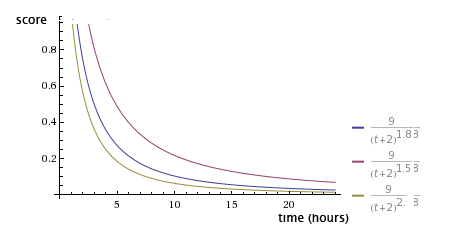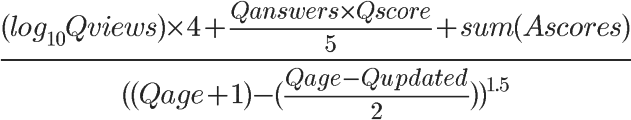# 【推荐算法工程师技术栈系列】推荐系统--热榜召回

## 排名公式

### Hacker News(帖子投票)

$score = \frac{(P-1)}{(T+2)^G}$

$其中P = 文章获得的票数( -1 是去掉文章提交人的票)$
$T = 从文章提交至今的时间(小时)$
$G = 比重，news.arc里缺省值是1.8$### Reddit(帖子赞踩)

$f(t_s, y, z)=\log_{10} z+\frac{y t_{s}}{45000}$

$其中t_{s}是发帖时间据网站成立的时间$
$y 是一个符号变量，表示对文章的总体看法。如果赞成票居多，y就是 +1；如果反对票居多，y就是-1；如果赞成票和反对票相等，y就是0。$
$z 表示赞成票超过反对票的数量。如果赞成票少于或等于反对票，那么z就等于1。$

结论就是，Reddit 的排名，基本上由发帖时间决定，超级受欢迎的文章会排在最前面，一般性受欢迎的文章、有争议的文章都不会很靠前。这决定了 Reddit 是一个符合大众口味的社区，不是一个很激进、可以展示少数派想法的地方。

### Stack Overflow(问题的得分、回答的数目和该问题的浏览次数)1）Qviews（问题的浏览次数）

首先，Qscore（问题得分）= 赞成票-反对票。如果某个问题越受到好评，排名自然应该越靠前。

3）Ascores（回答得分）
一般来说，"回答"比"问题"更有意义。这一项的得分越高，就代表回答的质量越高。
但是我感觉，简单加总的设计还不够全面。这里有两个问题。首先，一个正确的回答胜过一百个无用的回答，但是，简单加总会导致，1个得分为 100 的回答与 100 个得分为 1 的回答，总得分相同。其次，由于得分会出现负值，因此那些特别差的回答，会拉低正确回答的得分。

4）Qage（距离问题发表的时间）和 Qupdated（距离最后一个回答的时间）
改写一下，可以看得更清楚：
Qage 和 Qupdated 的单位都是秒。如果一个问题的存在时间越久，或者距离上一次回答的时间越久，Qage 和 Qupdated 的值就相应增大。
也就是说，随着时间流逝，这两个值都会越变越大，导致分母增大，因此总得分会越来越小。

５）总结
Stack Overflow 热点问题的排名，与参与度（Qviews 和 Qanswers）和质量（Qscore 和 Ascores）成正比，与时间（Qage 和 Qupdated）成反比。

### 牛顿冷却定律

$T=T_{0} e^{-\alpha\left(t-t_{0}\right)}$

• T(t)是温度T的关于时间t的函数。微积分知识告诉我们，温度变化（冷却）的速率就是温度函数的导数T'(t)。
• $T_{0}$是文章的初始温度，比如100
• $t-t_{0}为距离创建时间的小时数$
• 常数α（α>0）表示冷却系数；如果你想放慢"热文排名"的更新率，"冷却系数"就取一个较小的值，否则就取一个较大的值。### 威尔逊区间

$\frac{p+\frac{1}{2 n} z_{1-\alpha / 2}^{2} \pm z_{1-\alpha / 2} \sqrt{\frac{p(1-p)}{n}+\frac{z_{1-\alpha / 2}^{2}}{4 n^{2}}}}{1+\frac{1}{n} z_{1-\alpha / 2}^{2}}$

• $p表示样本的"赞成票比例"$
• n表示样本的大小
• $z_{1-\alpha / 2}$表示对应某个置信水平的z统计量，这是一个常数，可以通过查表或统计软件包得到。一般情况下，在95%的置信水平下，z统计量的值为1.96。

### IMDB算法（贝叶斯算法,电影评分）

$WR = \frac{v}{v+m} * R + \frac{m}{v+m} * C$

• WR， 加权得分（weighted rating）
• R， 该电影的用户投票的平均得分（Rating）
• m， 排名前 250 名的电影的最低投票数
• C， 所有电影的平均得分

### 梅西法（BCS体育赛事）

$X^T Xr=X^T y$

• $X^TX构造为如下矩阵，对角元素就是队伍i完成的比赛场数;i,j每有一个比赛/评价，非对角元素ij和ji位置的值减一。$
• $针对X^Ty的第i个元素就是队伍i所有比赛获得的分差之和。$
这里简单分析下$X^TX$是一个n阶对称方阵，也是一个对角阵，并且每一列线性相关。
为了让r有唯一解，往往需要给$X^TX$$X^Ty$增加一行0，表示每个队伍所有评分总和为0.

### 科利法（Netflix电影评分）

$Cr=b$

• 𝐶的主对角线上为队伍i完成的比赛场数+2，i,j每有一个比赛/评价，非对角元素ij和ji位置的值减一。
• $b_{i}=1+0.5(w_{i}-l_{i})$,其中$𝑤_𝑖$,$l_𝑖$ 为获胜场数和落败场数。

1.热榜涉及需要考虑的几点因素

2.热榜的几种分类

## 附录

posted @ 2020-03-14 22:06  混沌战神阿瑞斯  阅读(345)  评论(0编辑  收藏